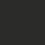# How to show two lengths are equal

There are many ways to show two segments are of equal length. Let us first discuss such problems. There are too many theorems and results which can be used, but let us just list a few more common techniques:

• Using congruent triangles;

• Using a third line segment;

• Using isosceles triangles;

• Using parallelograms;

• Circle Properties: Chords of equal length are of the same distance from the center of the circle, chords subtended by the same angle at the center of the circle are of the same length, etc;

• If a line parallel to one side of a triangle bisects a second side, it also bisects the third side;

• Comparing the lengths with multiples or $\frac{1}{2}$, $\frac{1}{3}$ ... of another length.

Exercises

$1$. Construct squares $ABEF$ and $ACGH$ externally to the sides $AB$ and $AC$ respective of triangle $ABC$. Let $D$ be the foot of the perpendicular from $A$ to $BC$, and let $AD$ intersect $FH$ at $M$. Show that $FM=MH$.

$2$. In $\triangle{ABC}$, $AB=AC$. Let $D$ be a point on $AB$ and $E$ be a point on $AC$ extended such that $BD=CE$. Let $DE$ intersect $BC$ at $F$. Show that $DF=FE$.

$3$. Let $E$ and $F$ be the midpoints on the sides $BC$ and $AD$ respectively of a parallelogram $ABCD$. Show that $BF$ and $DE$ trisect $AC$.

$4$. Let $ABC$ be a triangle. Construct equilateral triangles $ABD$ and $ACE$ externally to sides $AB$ and $AC$ respectively. Let $F$ be the point such that $ADFE$ is a parallelogram. Show that $\triangle{FBC}$ is equilateral.

$5$. Let $BD$ and $CE$ be the altitudes of a triangle $ABC$. Let $F$ be the midpoint of $BC$ and $G$ be on $DE$ such that $DE \perp FG$. Show that $DG=GE$.Note by Victor Loh
6 years, 11 months ago

This discussion board is a place to discuss our Daily Challenges and the math and science related to those challenges. Explanations are more than just a solution — they should explain the steps and thinking strategies that you used to obtain the solution. Comments should further the discussion of math and science.

When posting on Brilliant:

• Use the emojis to react to an explanation, whether you're congratulating a job well done , or just really confused .
• Ask specific questions about the challenge or the steps in somebody's explanation. Well-posed questions can add a lot to the discussion, but posting "I don't understand!" doesn't help anyone.
• Try to contribute something new to the discussion, whether it is an extension, generalization or other idea related to the challenge.

MarkdownAppears as
*italics* or _italics_ italics
**bold** or __bold__ bold
- bulleted- list
• bulleted
• list
1. numbered2. list
1. numbered
2. list
Note: you must add a full line of space before and after lists for them to show up correctly
paragraph 1paragraph 2

paragraph 1

paragraph 2

[example link](https://brilliant.org)example link
> This is a quote
This is a quote
    # I indented these lines
# 4 spaces, and now they show
# up as a code block.

print "hello world"
# I indented these lines
# 4 spaces, and now they show
# up as a code block.

print "hello world"
MathAppears as
Remember to wrap math in $$ ... $$ or $ ... $ to ensure proper formatting.
2 \times 3 $2 \times 3$
2^{34} $2^{34}$
a_{i-1} $a_{i-1}$
\frac{2}{3} $\frac{2}{3}$
\sqrt{2} $\sqrt{2}$
\sum_{i=1}^3 $\sum_{i=1}^3$
\sin \theta $\sin \theta$
\boxed{123} $\boxed{123}$